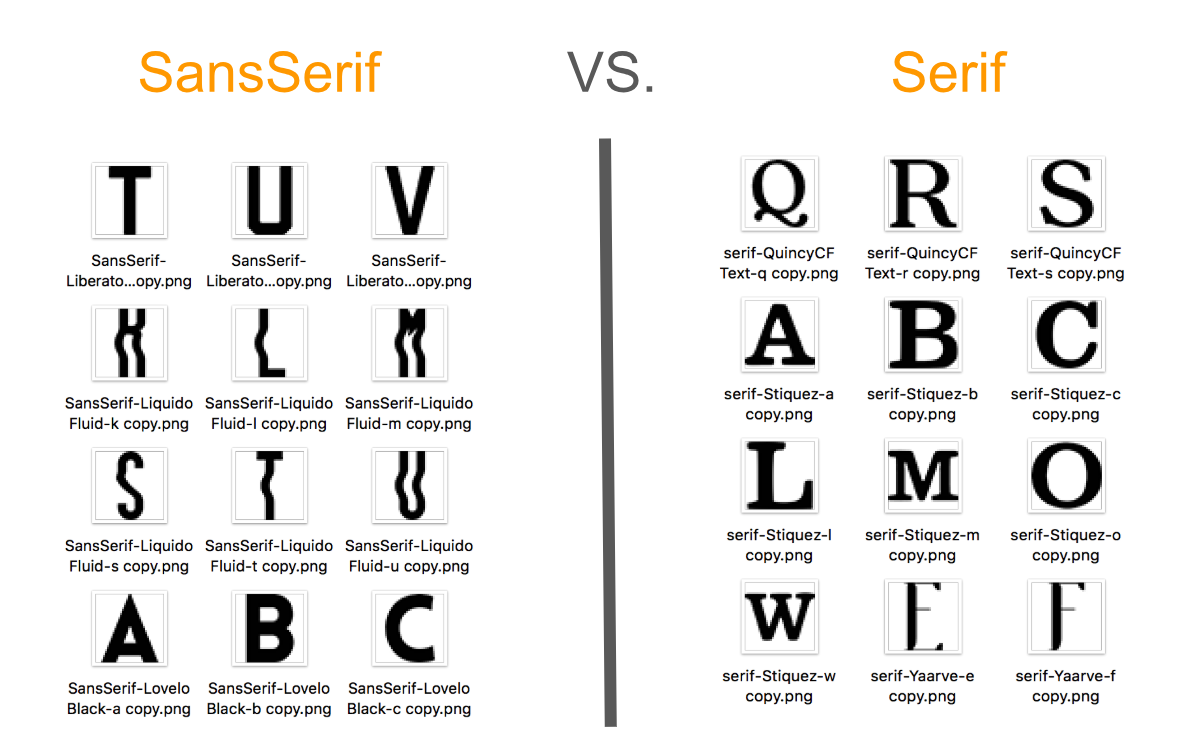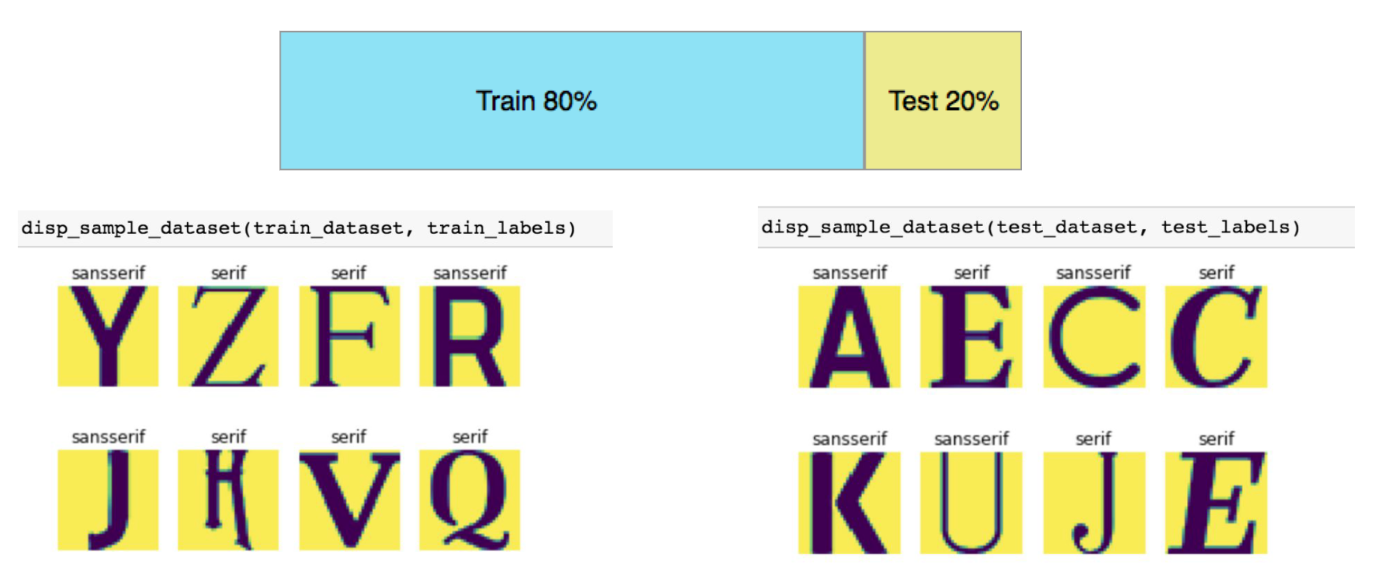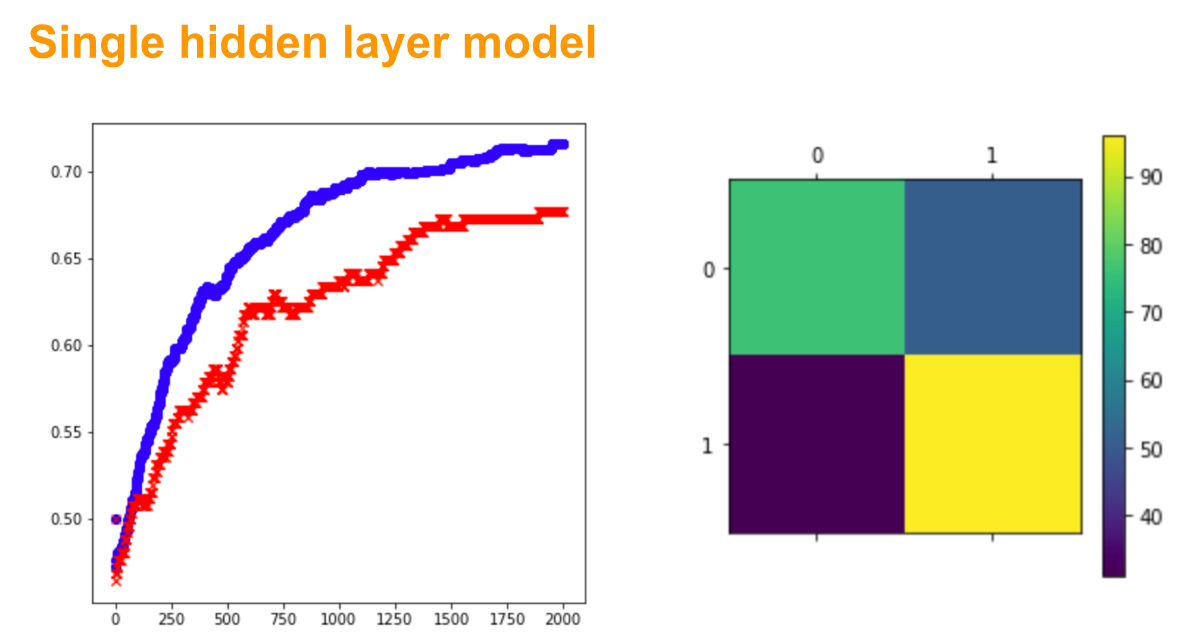# How to Build Deep Learning Models for Font Classification with Tensorflow: CNN, Deeper CNN, Hidden Layers Models

You will learn how to build and train decent deep learning models for classification problem with a few lines of code in TensorFlow. The 5 models covered in this article are:

• Logistic regression model
• The single hidden layer model
• Multiple hidden layers model
• Deep CNN with convolutional layer and pooling layer
• Deeper CNN with 2 convolutional layers and 2 pooling layers

The following methods have been used for achieving better performance in this project:

• Gradient descent optimizer
• RELU activation function
• Learning rate decay
• One hot encoding
• Cross entropy
• Dropout

There are images of letters in fonts Sans Serif and Serif. The goal is to identify fonts based on one specific image of a single letter. It is a straightforward classification problem.2 types of fonts

#### Data engineering

1. Convert images to grayscale with 36*36 pixels
2. Add labels to dataset: font SansSerif = 0, font Serif = 1
3. Shuffle and split the dataset into 2 datasets: train dataset (80%) and test dataset (20%)train dataset and test dataset

#### Logistic regression model

1. Model specification

2. Model training

Train accuracy: 0.718935

Test accuracy: 0.692913

#### Single hidden layer model

1. Model specification

2. Model trainingconfusion matrix

#### Multiple hidden layers model

1. Model specification

2. Model training

#### Deep CNN with convolutional layer and pooling layer

1. Model specification

2. Model training

#### Deeper CNN with 2 convolutional layers and 2 pooling layers

1. Model specification

2. Model training

#### Summary

Congrats! You just learn how to build and train 5 deep learning models for classification problems using Tensorflow.

One more thing about adding pooling layer is that because of the pooling, the image size is gradually shrinking. Early convolutional weights often train to detect simple edges, while successive convolutional layers combine those edges into gradually more complex shapes such as faces, cars, and even dogs.

Human learning is the beginning of Deep learning! Have fun.